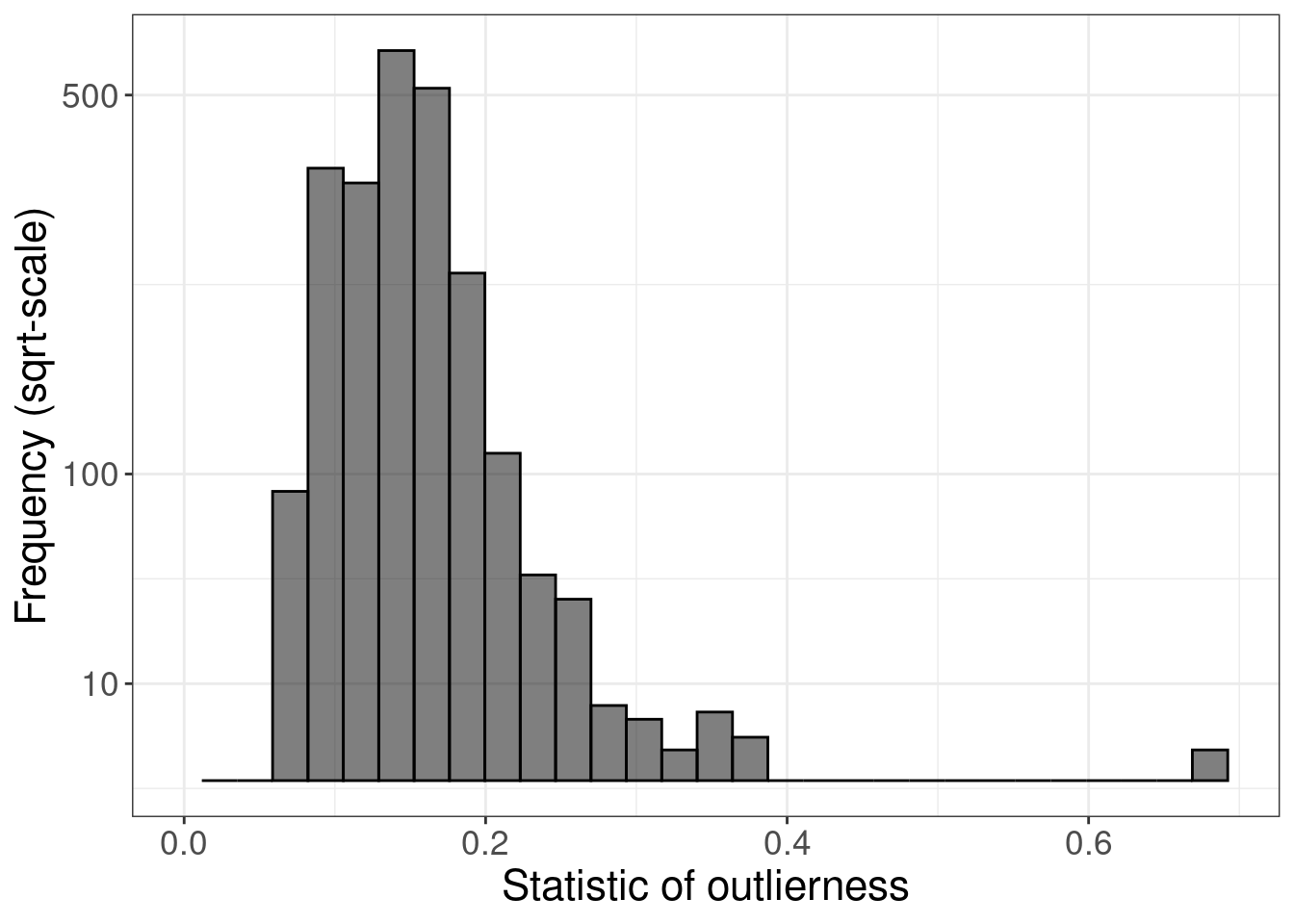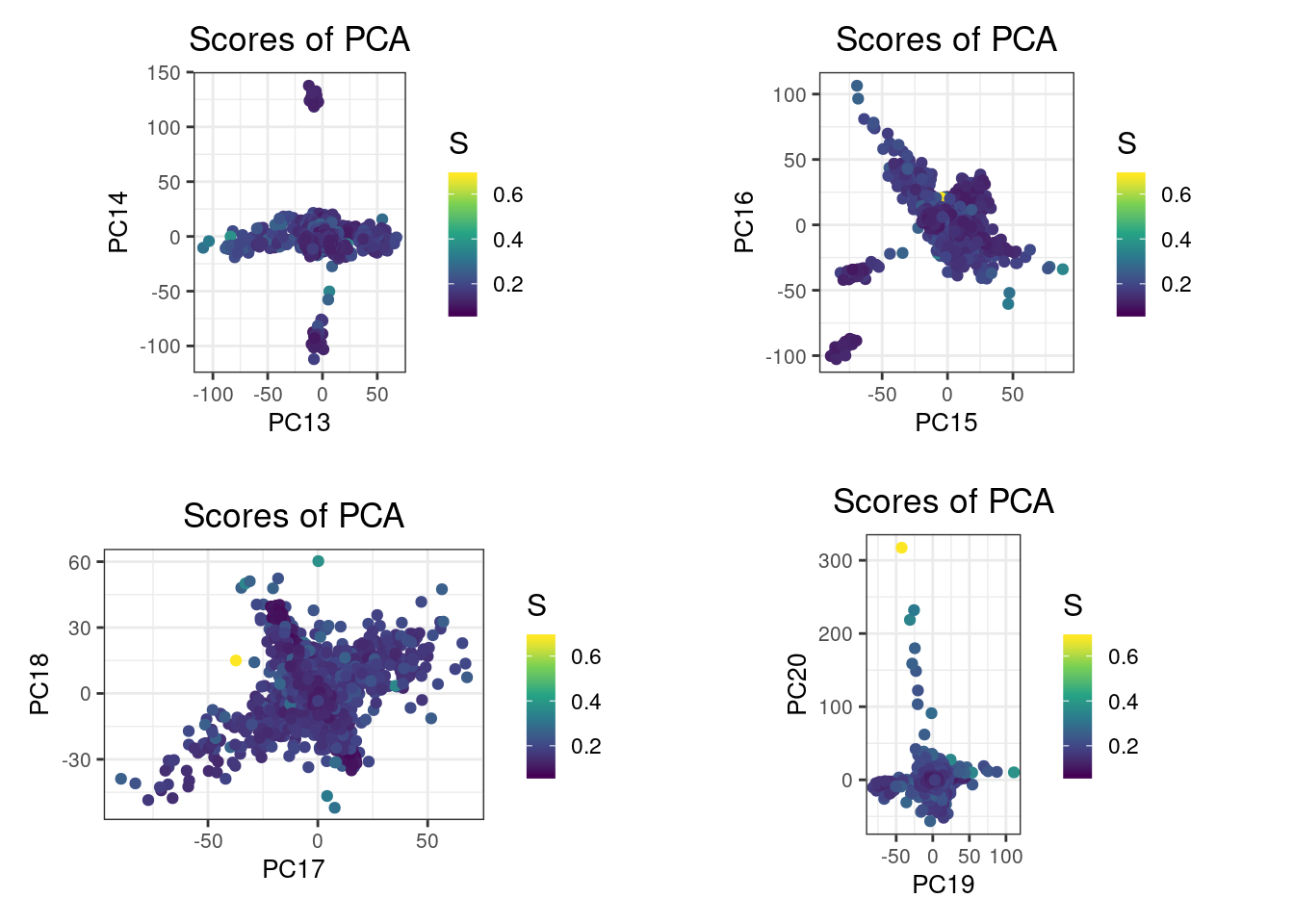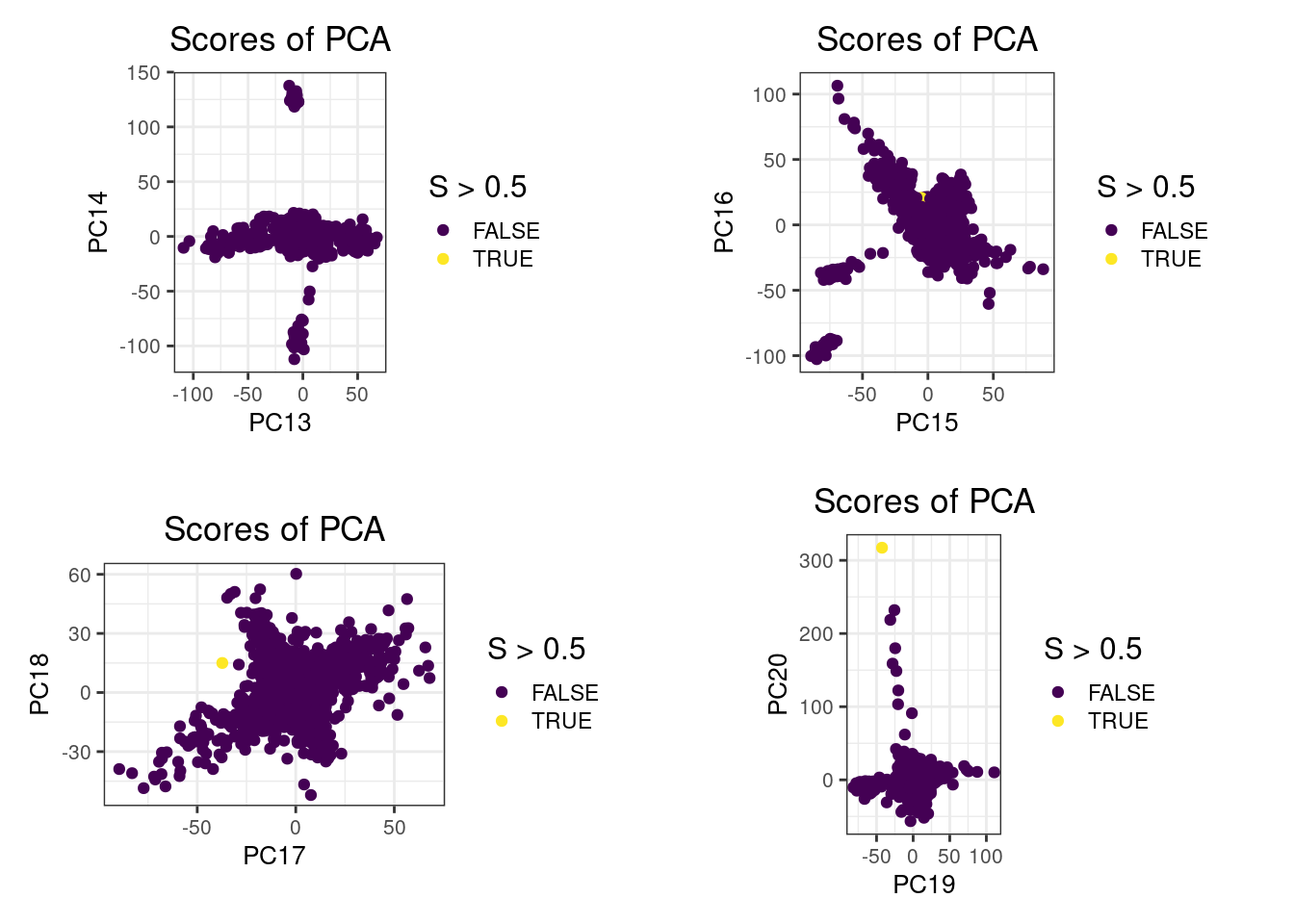``library(bigsnpr)``
``## Loading required package: bigstatsr``
``library(ggplot2)``

## Data

Let us use a subsetted version of the 1000 Genomes project data we provide. Some quality control has already been done; otherwise, you can use `snp_plinkQC()`.

``bedfile <- download_1000G("data")``

## Relatedness

First, let us detect all pairs of related individuals.

``````plink2 <- download_plink2("data")
bedfile.in = bedfile,
thr.king = 2^-4.5,
make.bed = FALSE,
ncores = nb_cores()
)
str(rel)``````
``````## 'data.frame':    31 obs. of  8 variables:
##  \$ FID1   : int  0 0 0 0 0 0 0 0 0 0 ...
##  \$ ID1    : chr  "HG00120" "HG00240" "HG00542" "HG00595" ...
##  \$ FID2   : int  0 0 0 0 0 0 0 0 0 0 ...
##  \$ ID2    : chr  "HG00116" "HG00238" "HG00475" "HG00584" ...
##  \$ NSNP   : int  1664852 1664852 1664852 1664852 1664852 1664852 1664852 1664852 1664852 1664852 ...
##  \$ HETHET : num  0.111 0.1105 0.1024 0.101 0.0992 ...
##  \$ IBS0   : num  0.0333 0.0367 0.0302 0.037 0.0367 ...
##  \$ KINSHIP: num  0.0821 0.068 0.0854 0.0541 0.0535 ...``````

## Principal Component Analysis (PCA)

We then compute PCA without using the related individuals. Function `bed_autoSVD()` should take care of Linkage Disequilibrium (LD).

``(obj.bed <- bed(bedfile))``
``## A 'bed' object with 2490 samples and 1664852 variants.``
``````ind.rel <- match(c(rel\$IID1, rel\$IID2), obj.bed\$fam\$sample.ID)  # /!\ use \$ID1 instead with old PLINK
ind.norel <- rows_along(obj.bed)[-ind.rel]

obj.svd <- bed_autoSVD(obj.bed, ind.row = ind.norel, k = 20,
ncores = nb_cores())``````
``````##
## Phase of clumping (on MAC) at r^2 > 0.2.. keep 392665 variants.
## Discarding 0 variant with MAC < 10.
##
## Iteration 1:
## Computing SVD..
## 679 outlier variants detected..
## 7 long-range LD regions detected..
##
## Iteration 2:
## Computing SVD..
## 0 outlier variant detected..
##
## Converged!``````

## Outlier sample detection

Then, we look at if there are individual outliers.

``````prob <- bigutilsr::prob_dist(obj.svd\$u, ncores = nb_cores())
S <- prob\$dist.self / sqrt(prob\$dist.nn)

ggplot() +
geom_histogram(aes(S), color = "#000000", fill = "#000000", alpha = 0.5) +
scale_x_continuous(breaks = 0:5 / 5, limits = c(0, NA)) +
scale_y_sqrt(breaks = c(10, 100, 500)) +
theme_bigstatsr() +
labs(x = "Statistic of outlierness", y = "Frequency (sqrt-scale)")``````
``## `stat_bin()` using `bins = 30`. Pick better value with `binwidth`.``
``## Warning: Removed 1 rows containing missing values (geom_bar).````````plot_grid(plotlist = lapply(7:10, function(k) {
plot(obj.svd, type = "scores", scores = 2 * k - 1:0, coeff = 0.6) +
aes(color = S) +
scale_colour_viridis_c()
}), scale = 0.95)````````````plot_grid(plotlist = lapply(7:10, function(k) {
plot(obj.svd, type = "scores", scores = 2 * k - 1:0, coeff = 0.6) +
aes(color = S > 0.5) +  # threshold based on histogram
scale_colour_viridis_d()
}), scale = 0.95)``````## PCA without outlier

We recompute PCA without outliers, starting with the previous set of variants kept (we can therefore skip the initial clumping step).

``````ind.row <- ind.norel[S < 0.5]
ind.col <- attr(obj.svd, "subset")
obj.svd2 <- bed_autoSVD(obj.bed, ind.row = ind.row,
ind.col = ind.col, thr.r2 = NA,
k = 20, ncores = nb_cores())``````
``````##
## Skipping clumping.
## Discarding 0 variant with MAC < 10.
##
## Iteration 1:
## Computing SVD..
## 22 outlier variants detected..
## 1 long-range LD region detected..
##
## Iteration 2:
## Computing SVD..
## 0 outlier variant detected..
##
## Converged!``````

## Verification

``plot(obj.svd2)``